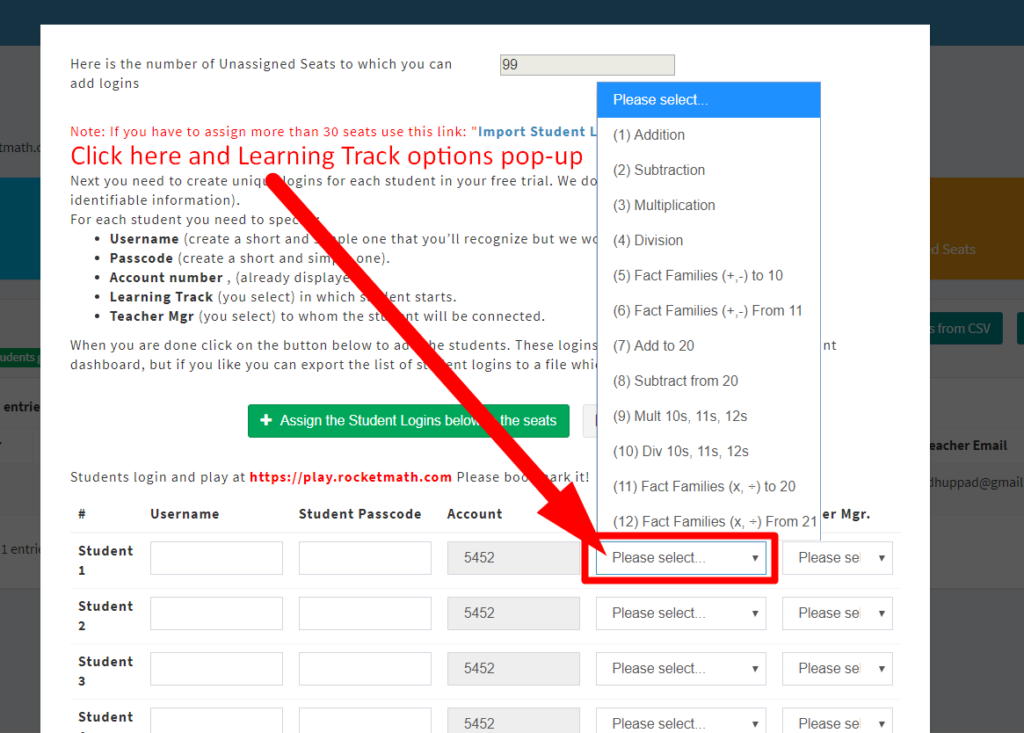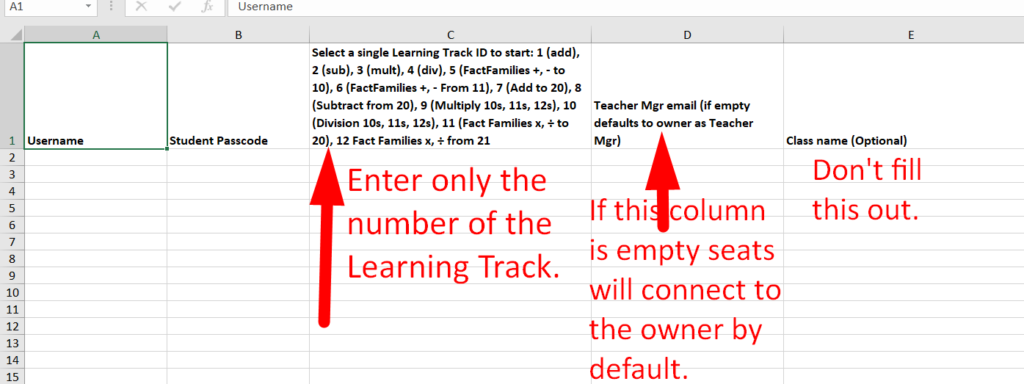3.A) How to choose (and assign) Learning Tracks

Choose from twelve Learning Tracks

In the Rocket Math Online Game every student needs to be started in one of the operations or Learning Tracks. A student’s Learning Track can be changed at any time**, but one must be chosen to begin with.

Entering up to 30 students on the pop-upIf you are entering the Student Login with the popup, you can use the pull down menu to select a learning track, for each student as illustrated. You can then assign a different learning track to each student.

Entering more than 30 students using a CSV fileIf you are entering the Student Login using the csv method of entry you’ll be using the template we provide.  In column C do NOT enter the names of the learning tracks.  You must enter a number for each Learning Track in column C. The template gives you the numbers at the top of column C.  The learning tracks are numbered as follows.

1. Addition 1s through 9s
2. Subtraction 1s through 9s
3. Multiplication 1s through 9s
4. Division 1s through 9s
5. Fact Families (+, -) to 10, ex.4+6, 6+4, 10-4, 10-6
6. Fact Families (+, -) from 11, ex. 5+6, 6+5, 11-6, 11-5.
7. Add to 20, example 13+4, 4+13,
8. Subtract from 20, example 15-3, 15-12,
9. Multiplication 10s-11s-12s,
10. Division 10s-11s-12s.
11. Fact Families (x, ÷) to 20, example 4×5, 5×4, 20÷4, 20÷5
12. Fact Families (x, ÷) from 21,example 3×7, 7×3, 21÷3, 21÷7

You can click this link to see a google document showing all the problems learned in each of the Learning Tracks. Click to see the problems in the tracks.

Considerations, or what to choose when?

Begin with the basics. The four basic operations are most important and typical expectations is one of those per grade level, so

• 3rd grade:  Multiplication, then go back to Addition and Subtraction,
• 4th grade and above:  Multiplication then Division, and then going back to get Addition and Subtraction if those haven’t been learned.

Fact Families (+, -) is another way to learn basic Addition and Subtraction Facts.

• 1st grade: (5) Fact Families (+, -) to 10.
• 2nd grade: (6) Fact Families (+, -) from 11.
• You can choose Fact Families instead of the basic addition and basic subtraction fact Learning Tracks.
• Optionally, Fact Families is also a good way to review for students who have already learned the basic addition and subtraction facts in first or second grade.

Fact Families (x, ÷) is another way to learn basic Multiplication and Division Facts

• 3rd grade:  (11) Fact Families (x, ÷) to 20.
• 4th grade  (12) Fact Families (x,÷) From 21.
• You can choose Fact Families instead of the basic multiplication and division fact Learning Tracks
• Optionally, Fact Families are useful as a review for students who have already learned the basic multiplication and division facts in 3rd or 4th grade.

Make sure your students have worked through the expected basic operations for their grade level BEFORE doing any of the optional Learning Tracks.

Optional Learning Tracks–for students who have finished the basics. (7) Add to 20 and (8) Subtract from 20 are additional problems that the Common Core feels should be committed to memory. They are composed of facts you can figure out if you know the basic 1s through 9s facts, but can be learned AFTER the basics are learned, if there is time in first or second grade. They should not be assigned until after the student has mastered the basic 1s through 9s addition and subtraction facts.

After students learn the basic 1s through 9s multiplication facts, if there is time, they can move on to 10s, 11s, 12s. After basic 1s through 9s division facts are learned (and all the other basic operations are learned) then the 10s, 11s, and 12s are a good use of time.

**See “How to change Learning Tracks” in the FAQs and Directions document.

This entry was posted in . Bookmark the permalink.